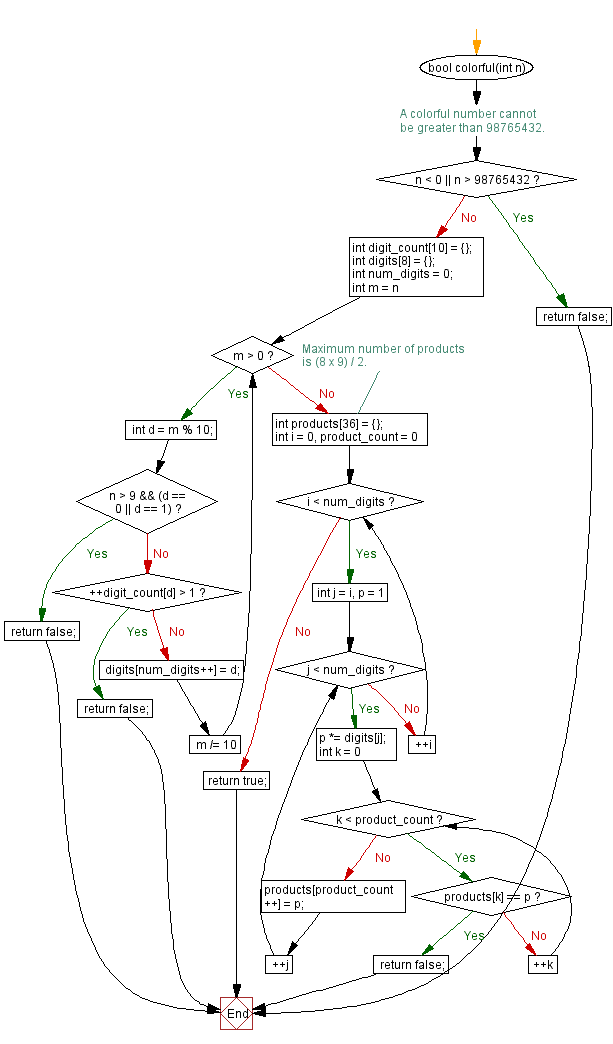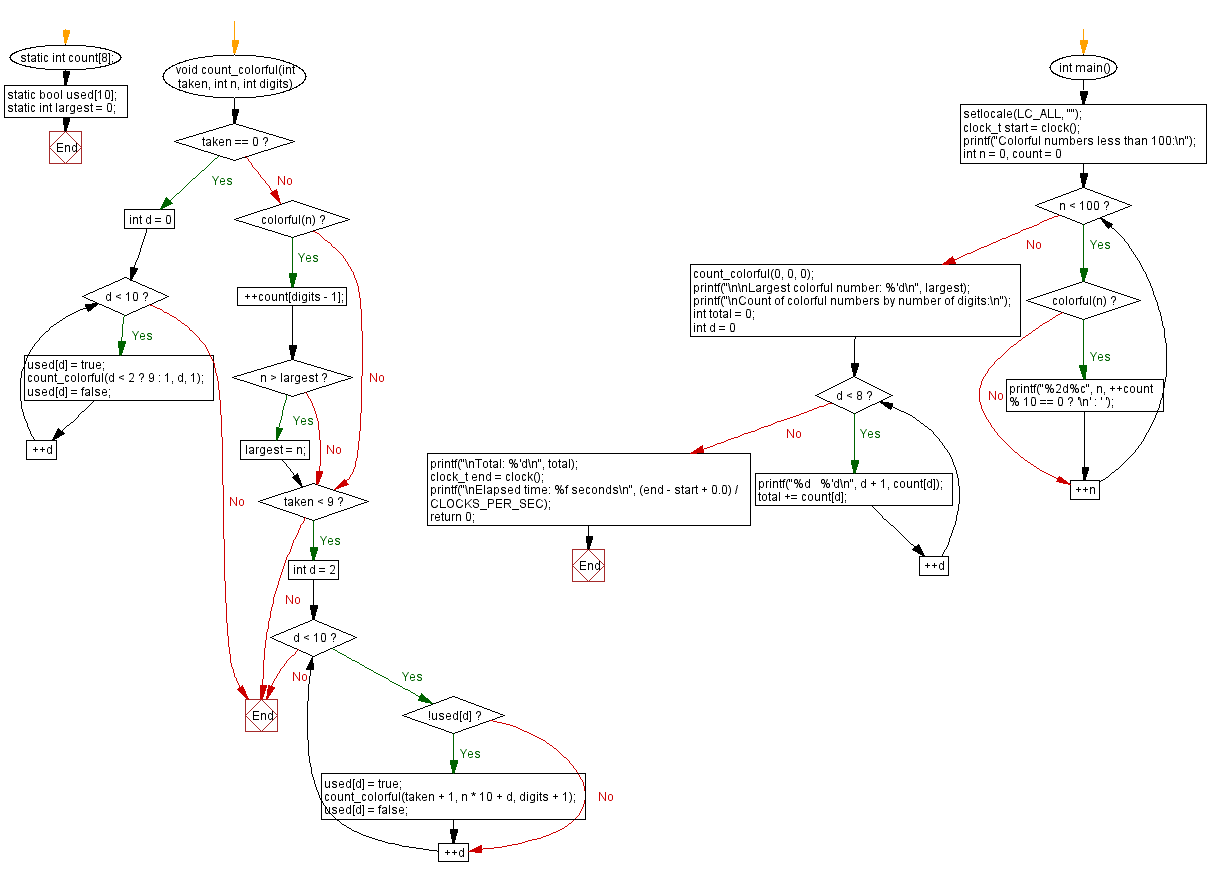﻿ C Program - Colorful numbers# C Exercises: Colorful numbers

## C Programming Challenges: Exercise-34 with Solution

A colorful number is a non-negative base 10 integer where the product of every sub group of consecutive digits is unique.
i.e.
24753 is a colorful number. 2, 4, 7, 5, 3, (2×4)8, (4×7)28, (7×5)35, (5×3)15, (2×4×7)56, (4×7×5)140, (7×5×3)105, (2×4×7×5)280, (4×7×5×3)420, (2×4×7×5×3)840
Every product is unique.
2346 is not a colorful number. 2, 3, 4, 6, (2×3)6, (3×4)12, (4×6)24, (2×3×4)48, (3×4×6)72, (2×3×4×6)14
The product 6 is repeated.
Single digit numbers are considered to be colorful. A colorful number larger than 9 cannot contain a repeated digit, the digit 0 or the digit 1. As a consequence, there is a firm upper limit for colorful numbers; no colorful number can have more than 8 digits.
Write a C program (subroutine, function, procedure, whatever it may be called in your language) to test if a number is a colorful number or not.
Use that routine to find all of the colorful numbers less than 100.
Use that routine to find the largest possible colorful number.

Sample Data:
Colorful numbers less than 100:
0 1 2 3 4 5 6 7 8 9
23 24 25 26 27 28 29 32 34 35
36 37 38 39 42 43 45 46 47 48
49 52 53 54 56 57 58 59 62 63
64 65 67 68 69 72 73 74 75 76
78 79 82 83 84 85 86 87 89 92
93 94 95 96 97 98

C Code:

``````//#Source shorturl.at/qvw79
#include <locale.h>
#include <stdbool.h>
#include <stdio.h>
#include <time.h>

bool colorful(int n) {
// A colorful number cannot be greater than 98765432.
if (n < 0 || n > 98765432)
return false;
int digit_count = {};
int digits = {};
int num_digits = 0;
for (int m = n; m > 0; m /= 10) {
int d = m % 10;
if (n > 9 && (d == 0 || d == 1))
return false;
if (++digit_count[d] > 1)
return false;
digits[num_digits++] = d;
}
// Maximum number of products is (8 x 9) / 2.
int products = {};
for (int i = 0, product_count = 0; i < num_digits; ++i) {
for (int j = i, p = 1; j < num_digits; ++j) {
p *= digits[j];
for (int k = 0; k < product_count; ++k) {
if (products[k] == p)
return false;
}
products[product_count++] = p;
}
}
return true;
}

static int count;
static bool used;
static int largest = 0;

void count_colorful(int taken, int n, int digits) {
if (taken == 0) {
for (int d = 0; d < 10; ++d) {
used[d] = true;
count_colorful(d < 2 ? 9 : 1, d, 1);
used[d] = false;
}
} else {
if (colorful(n)) {
++count[digits - 1];
if (n > largest)
largest = n;
}
if (taken < 9) {
for (int d = 2; d < 10; ++d) {
if (!used[d]) {
used[d] = true;
count_colorful(taken + 1, n * 10 + d, digits + 1);
used[d] = false;
}
}
}
}
}

int main() {
setlocale(LC_ALL, "");

clock_t start = clock();

printf("Colorful numbers less than 100:\n");
for (int n = 0, count = 0; n < 100; ++n) {
if (colorful(n))
printf("%2d%c", n, ++count % 10 == 0 ? '\n' : ' ');
}

count_colorful(0, 0, 0);
printf("\n\nLargest colorful number: %'d\n", largest);

printf("\nCount of colorful numbers by number of digits:\n");
int total = 0;
for (int d = 0; d < 8; ++d) {
printf("%d   %'d\n", d + 1, count[d]);
total += count[d];
}
printf("\nTotal: %'d\n", total);

clock_t end = clock();
printf("\nElapsed time: %f seconds\n",
(end - start + 0.0) / CLOCKS_PER_SEC);
return 0;
}
``````

Sample Output:

```Colorful numbers less than 100:
0  1  2  3  4  5  6  7  8  9
23 24 25 26 27 28 29 32 34 35
36 37 38 39 42 43 45 46 47 48
49 52 53 54 56 57 58 59 62 63
64 65 67 68 69 72 73 74 75 76
78 79 82 83 84 85 86 87 89 92
93 94 95 96 97 98

Largest colorful number: 98,746,253

Count of colorful numbers by number of digits:
1   10
2   56
3   328
4   1,540
5   5,514
6   13,956
7   21,596
8   14,256

Total: 57,256

Elapsed time: 0.036510 seconds
```

Flowchart:## C Programming Code Editor:

Contribute your code and comments through Disqus.

Previous C Programming Exercise: Attractive numbers up to 100.
Next C Programming Exercise: Calendar for any year.

What is the difficulty level of this exercise?

Test your Programming skills with w3resource's quiz.

﻿